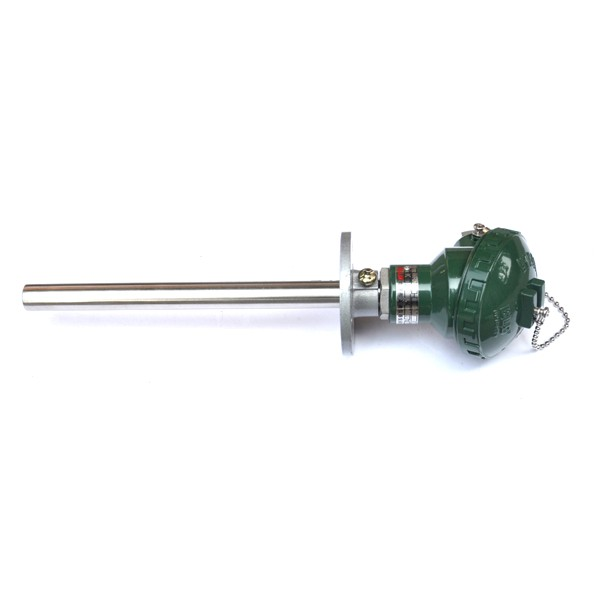【 微信扫码咨询 】

010-52882318

18612791968# 贴片高温热电偶

010-52882318

 产品型号 产品外形图（整图） 贴片部分外形图 WRNT-11 贴片外形尺寸图 贴片传感器默认规格参数（适用于250℃内的热电阻信号或0-600℃热电偶） 长度（L）=40mm 宽度（W）=20mm 厚度（D）=10mm 固定孔直径=8 mm 探头直径D=6 mm 探头长度：对于热电偶信号超过100℃时，就要将探头做到足够长，以便温度降到100℃内 备注说明 1、对于测量高于250℃的热电阻信号（Pt100、 Pt1000等），探头直径 D=8mm 贴片厚度=12mm 2、对于测量高于600℃的热电偶信号（K、E等），探头直径 D=8mm 贴片厚度=12mm 3、对于特殊的测温要求，可以定制贴片传感器的外形尺寸及固定孔和传感器的直径等参数

 贴片式温度传感器选型 WRNT-11 备注说明 口 K 9=指定 传感器类型 T口 0=0～80 1=-50～100 2=-50～150 3=-50～200 4=-50～300 5=-50～400 6=-50～500 7=-0～600 8=-0～800 9=指定 温度范围(℃) 温度高于500度只能选 热电偶 口 A=A级 B=B级 对于热电偶默为II级 传感器精度 C口 1=SUS321(默认) 4=SUS304 贴片材质 1 长度（L）=40mm 宽度（W）=20mm 厚度（D）=10mm 固定孔直径=8 mm 探头直径D=6 mm 贴片外形尺寸 默认规格1 2 长度（L）=40mm 宽度（W）=20mm 厚度（D）=12mm 固定孔直径=8 mm 探头直径D=8 mm 默认规格2 3 长度（L）=mm 宽度（W）=mm 厚度（D）=mm 固定孔直径=mm 探头直径D=mm 用户指定 贴片外形尺寸 I 1=50mm 2=100 3=150mm 4=200 5=300 6=500 7=1000 9=指定 探头长度 指露在贴片外面部分的长度 L口 1=100 2=200 3=300 5=500 10=1000 30=3000 50=5000 9=指定 引线长度(mm) X口 3=三线制 4=四线制 对于热电偶为两线 引出线采用线制 口 1=聚氯乙烯PVC（-20～80℃） 2=聚氨酯TPU （-50～100℃） 3=硅橡胶 （-60～200℃） 4=四氟绕包（-50～250℃） 5=云母绕包 （-80～500℃） 引线材质 “（）”内为引线常用温度范围 口 0=无屏蔽层（默认） 1=有屏蔽层 是否配屏蔽层 口 0=无护套 1=有护套 线缆护套 口 0=无 1=镀锡 3=压M4端子 9=指定 导线末端要求 Q 0=无 9=指定 特殊要求

 产品型号 产品外形图（整图） 贴片部分外形图 WRNT-11 贴片外形尺寸图 贴片传感器默认规格参数（适用于250℃内的热电阻信号或0-600℃热电偶） 长度（L）=40mm 宽度（W）=20mm 厚度（D）=10mm 固定孔直径=8 mm 探头直径D=6 mm 探头长度：对于热电偶信号超过100℃时，就要将探头做到足够长，以便温度降到100℃内 备注说明 1、对于测量高于250℃的热电阻信号（Pt100、 Pt1000等），探头直径 D=8mm 贴片厚度=12mm 2、对于测量高于600℃的热电偶信号（K、E等），探头直径 D=8mm 贴片厚度=12mm 3、对于特殊的测温要求，可以定制贴片传感器的外形尺寸及固定孔和传感器的直径等参数

 贴片式温度传感器选型 WRNT-11 备注说明 口 K 9=指定 传感器类型 T口 0=0～80 1=-50～100 2=-50～150 3=-50～200 4=-50～300 5=-50～400 6=-50～500 7=-0～600 8=-0～800 9=指定 温度范围(℃) 温度高于500度只能选 热电偶 口 A=A级 B=B级 对于热电偶默为II级 传感器精度 C口 1=SUS321(默认) 4=SUS304 贴片材质 1 长度（L）=40mm 宽度（W）=20mm 厚度（D）=10mm 固定孔直径=8 mm 探头直径D=6 mm 贴片外形尺寸 默认规格1 2 长度（L）=40mm 宽度（W）=20mm 厚度（D）=12mm 固定孔直径=8 mm 探头直径D=8 mm 默认规格2 3 长度（L）=mm 宽度（W）=mm 厚度（D）=mm 固定孔直径=mm 探头直径D=mm 用户指定 贴片外形尺寸 I 1=50mm 2=100 3=150mm 4=200 5=300 6=500 7=1000 9=指定 探头长度 指露在贴片外面部分的长度 L口 1=100 2=200 3=300 5=500 10=1000 30=3000 50=5000 9=指定 引线长度(mm) X口 3=三线制 4=四线制 对于热电偶为两线 引出线采用线制 口 1=聚氯乙烯PVC（-20～80℃） 2=聚氨酯TPU （-50～100℃） 3=硅橡胶 （-60～200℃） 4=四氟绕包（-50～250℃） 5=云母绕包 （-80～500℃） 引线材质 “（）”内为引线常用温度范围 口 0=无屏蔽层（默认） 1=有屏蔽层 是否配屏蔽层 口 0=无护套 1=有护套 线缆护套 口 0=无 1=镀锡 3=压M4端子 9=指定 导线末端要求 Q 0=无 9=指定 特殊要求

18612791968

010-52882318

##### 二维码